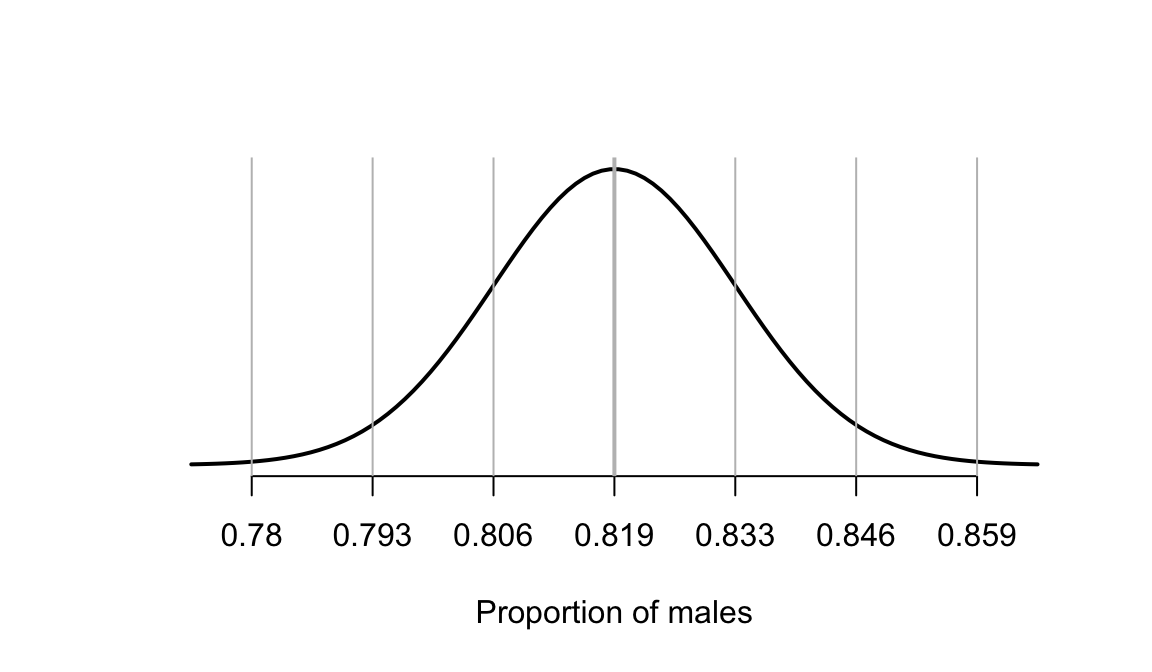## D.19 Answers: CIs for one proportion

Answers to exercises in Sect. 20.11.

Answer to Exercise 20.1: $$\hat{p} = 2182/6882 = 0.317059$$ and $$n=6882$$. So:

$\text{s.e.}(\hat{p}) = \sqrt{ \frac{0.317059 \times(1 - 0.317059)}{6882} } = 0.005609244.$ The CI is $$0.317059 \pm (2\times 0.005609244)$$, or $$0.317059\pm 0.01121849$$.

Rounding sensibly: $$0.317\pm 0.011$$ (notice we keep lots of decimal places in the working, but round the final answer).
Answer to Exercise 20.2: $$\hat{p} = 8/154 = 0.05194805$$; $$\text{s.e.}(\hat{p}) = 0.0017833$$; approximate 95% CI is $$0.05194 \pm (2 \times 0.0017833)$$, or $$0.0519\pm 0.0358$$, equivalent to 0.016 to 0.088. The CI is statistically valid.
Answer to Exercise 20.3: Use $$\hat{p} = 708/864 = 0.8194444$$ and $$n=864$$. Standard error: $$\text{s.e.}(\hat{p}) = 0.01308604$$; approximate 95% CI is $$0.8194444 \pm (2\times 0.01308604)$$. The CI is statistically valid.FIGURE D.6: The sampling distribution of the proportion of males in samples of 864 people with hiccups

Answer to Exercise 20.4: 1. Approximately $$n = 1/(0.05^2) = 400$$. 2. Approximately $$n = 1/(0.025^2) = 1600$$. 3. To halve the width of the interval, four times as many people are needed.

Answer to Exercise 20.5: After 3000 hours: $$\hat{p} = 0.2143$$; $$\text{s.e.}(\hat{p}) = 0.06331$$. The CI is from 0.088 to 0.341. The statistical validity conditions are satisfied.

After 400 hours: $$\hat{p} = 0$$; $$\text{s.e.}(\hat{p}) = 0$$. The CI is from 0 to 0: clearly silly (implies no sampling variation). This is because the statistical validity conditions are not satisfied.